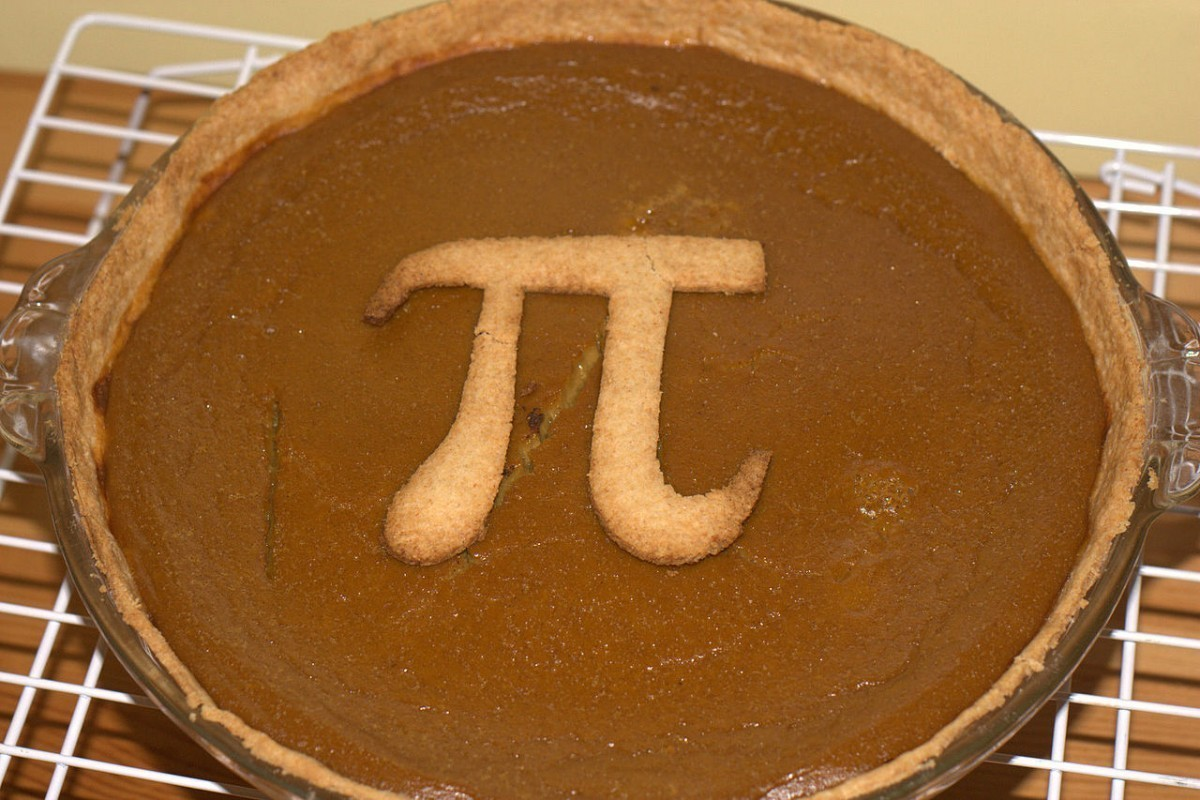# Cosmic Pi

In Mathematics by Brian Koberlein3 Comments

Today is March 14, which many celebrate as Pi Day since the month and day mark 3.14, which is approximately pi.It is also Albert Einstein’s birthday, so it seems fitting to ask whether pi can exist in a universe as Einstein described it.  Just for fun, I’m going to outline why the answer is no, and then explain why that answer is wrong.

The value of pi (3.14159…) is defined as the ratio of the circumference of a circle to its diameter.  But in a physical universe where (as Einstein demonstrated) space is curved, the ratio of circumference to diameter isn’t pi.  For example, if you drew a circle around the Earth, the ratio of circumference to diameter would actually be a little less than pi.  This is because the mass of the Earth curves space around it, making the diameter of your circle a bit longer than it should be.

This is actually a way you could define a region of space as being curved.  Draw a circle around a region of space, find the ratio of circumference to diameter, and if the value is less than pi then that region of space is curved.  The smaller the ratio, the more strongly that region of space is curved.  If you drew a circle around a black hole, its diameter would be infinite, so the ratio would be zero.

So if we define pi as the ratio of circle’s circumference to diameter in physical space, then pi would actually have lots of values depending the curvature of space around the circle, and none of them would be 3.14159…

Of course that isn’t how pi is defined.  The circumference/diameter definition applies for a mathematically ideal circle, where space isn’t curved.  It can also be defined in other ways, such as an infinite series pi = 4 – 4/3 + 4/5 – 4/7 + …  The geometry definition is just a simple (and perhaps the oldest) one. The key point is that pi is a mathematical concept, not a physical one.

So even though physical space is actually curved, pi still exists and has the value we all know and love.  So celebrate the day, because it is a perfect excuse to have a slice of pie.

1.2.3.